# Regular

All angles are equal and all sides are equal – everything equal.Regularly every day she measured the length of her plant. It was always the same.

NOTE:

Regular polygon – all angles are equal and all sides are equal on this many-sided shape.

An irregular polygon is the opposite, i.e. not regular.

Example 1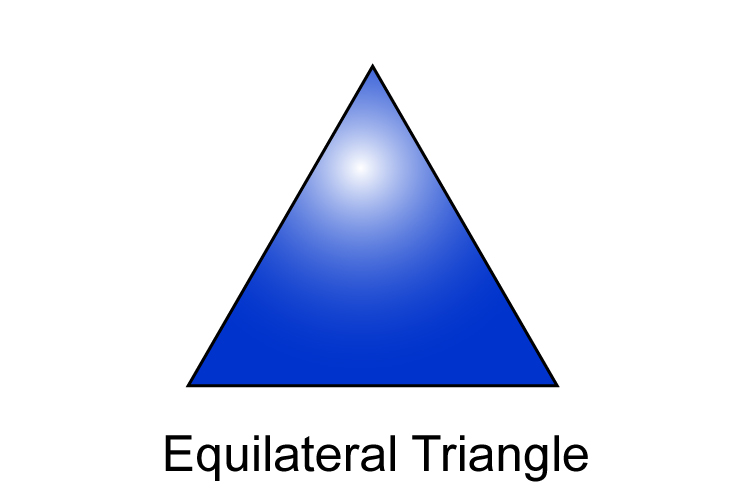Each internal angle between the sides of an equilateral triangle is 60° and each side is equal. This can also be described as a regular triangle.

Example 2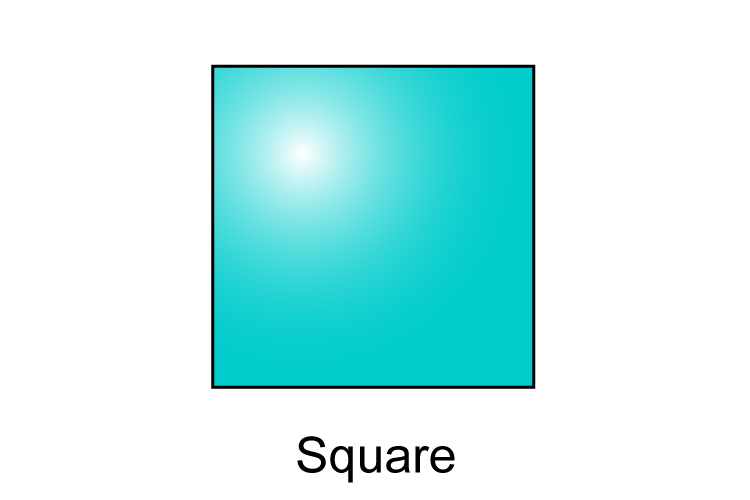Each internal angle between the sides of a square is 90°. This shape has equal sides and is a regular polygon.

Example 3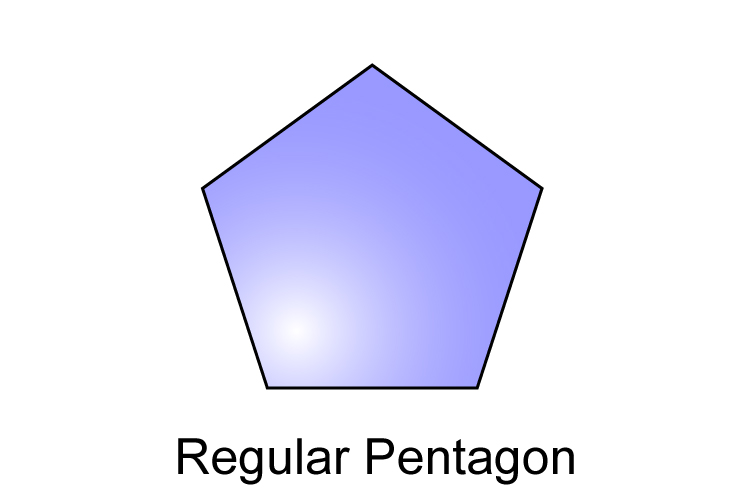The internal angle between the sides of a regular pentagon is 108°.

Example 4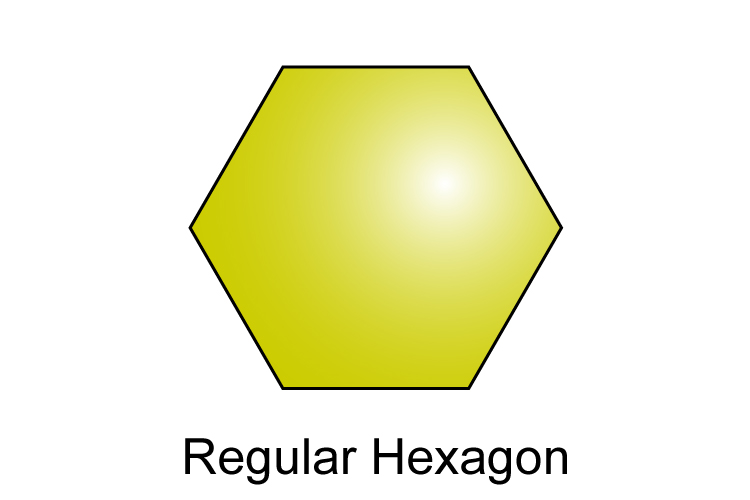The internal angle between the sides of a regular hexagon is 120°.

Example 5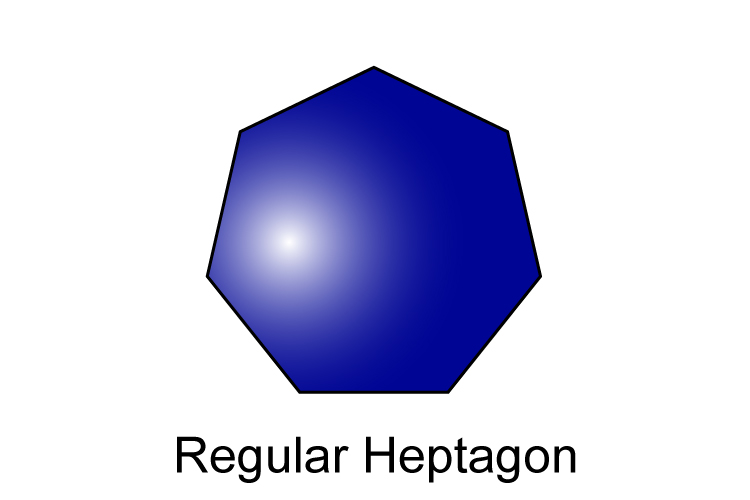The internal angle between the sides of a regular heptagon is 128°.

Example 6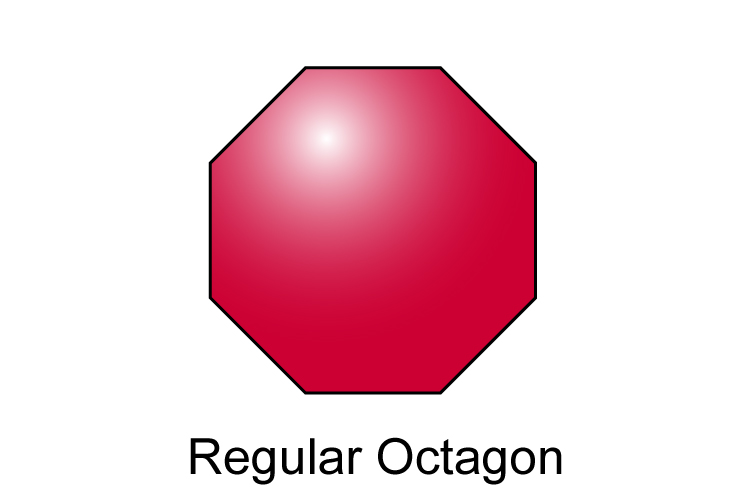The internal angle between the sides of a regular octagon is 135°.